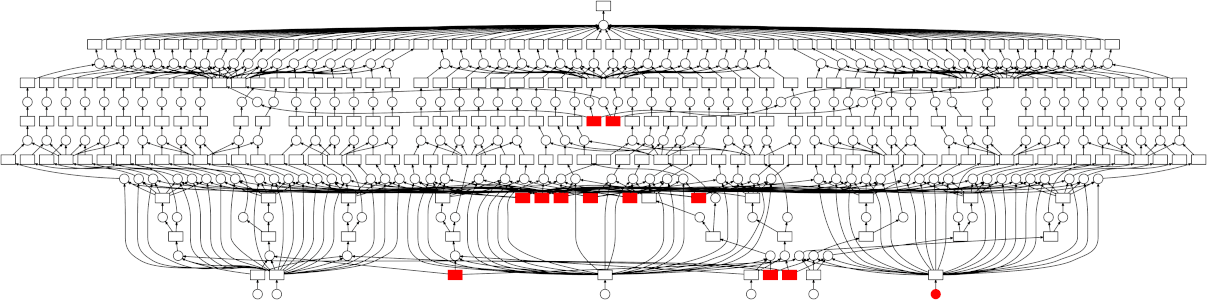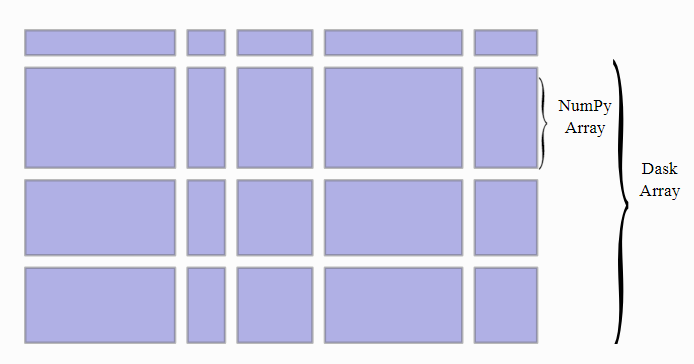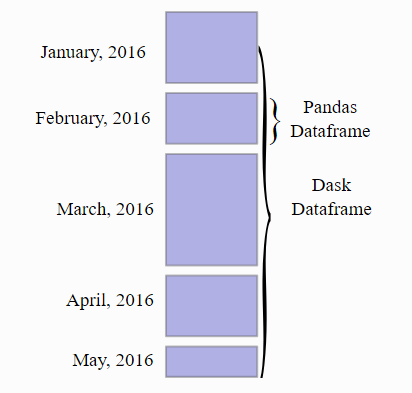This is the fourth post in a series I am writing. All posts are here:

1. Speed Up your Algorithms Part 1 — PyTorch
2. Speed Up your Algorithms Part 2 — Numba
3. Speed Up your Algorithms Part 3 — Parallelization

And these goes with Jupyter Notebooks available here:

Index

```NOTE:This post goes with Jupyter Notebook available in my Repo on Github:[SpeedUpYourAlgorithms-Dask]and on Kaggle:[SpeedUpYourAlgorithms-Dask]
```

1. Introduction ^

With increasing need for parallelization of Machine Learning algorithms, because of exponential increase in data sizes and even model sizes, it would have been really helpful if we had a tool which could help us parallelize our `Pandas`‘s DataFrame handling, which could parallilize our `Numpy`‘s computations, and even parallelize our Machine Learning algorithms (maybe algorithms from `sklearn` and `tensorflow`), efficiently, effectively and without much hassle.

But such a library does exist, and its name is `Dask`. `Dask` is a parallel computing library which doesn’t just help parallelize existing Machine Learning tools ( `Pandas` and`Numpy`)[i.e. using High Level Collection], but also helps parallelize low level tasks/functions and can handle complex interactions between these functions by making a tasks’ graph.[i.e. using Low Level Schedulers] This is similar to Threading or multiprocessing modules of Python.

They also have a separate Machine Learning library, `dask-ml`, which has integration with existing libraries such as `sklearn`, `xgboost` and `tensorflow`.

`Dask` parallelizes tasks given to it by making a graph of interactions between the tasks. It will be really helpful to visualize what you are doing by using `Dask`‘s `.visualize()` method which is available with all of its data types and with complex chain of tasks you compute. This method will output a graph of your tasks, and if your tasks have many nodes at each level(i.e. your tasks chain structure have many independent tasks at many levels, such as parallelizable task on chunks of data), then `Dask` will be able to parallelize them.2. Data Types ^Photo by Kai Oberhäuser on Unsplash

Each data type in `Dask` provides a distributed version of existing data types, such as `DataFrame` from `Pandas`, `ndarray`‘s from `numpy`, and `list` from `Python`. These data types can be larger than your memory, `Dask` will run computations on your data parallel(y) in `Blocked` manner. `Blocked` in the sense that they perform large computations by performing many small computations, i.e. in blocks, and number of blocks are total number of `chunks`.

a) Array:Many Numpy arrays in a grid as Dask Array

Dask Array operates on very large arrays, by dividing them into chunks and executing those blocks parallely. It has many of numpy methods available which you can use to get speedup. But some of them are not implemented.

Dask Array can read from any array like structure given it supports numpy like slicing and has `.shape` property by using `dask.array.from_array` method. It can also read from `.npy` and `.zarr` files.

```import dask.array as daimport numpy as np
```
```arr = numpy.random.randint(1, 1000, (10000, 10000))
```
```darr = da.from_array(arr, chunks=(1000, 1000))# It will make chunks, each of size (1000, 1000)darr.npartitioins# 100
```

It can be used when your arrays are really heavy (i.e. they won’t fit into memory) and `numpy` won’t be able to do anything about that. So, `Dask` divides them into chunks of arrays and operate on them in parallel for you.

Now, `Dask` does lazy evaluation of every method. So, to actually compute the value of a function, you have to use `.compute()` method. It will compute the result parallely in blocks, parallelizing every independent task at that time.

```result = darr.compute()
```

b) DataFrame:5 Pandas’ DataFrames each providing monthly data (can be from diff files) in one Dask DataFrame

Similar to `Dask Arrays`, `Dask DataFrame`s parallelize computation on very large Data Files, which won’t fit on memory, by dividing files into chunks and computing functions to those blocks parallely.

```import dask.dataframe as dddf = dd.read_csv("BigFile(s).csv", chunksize=50000)
```

Now you can apply/use most of the functions available in `pandas` library and apply it here.

```agg = df.groupby(["column"]).aggregate(["sum", "mean", "max", "min"])agg.columns = new_column_names # see in notebookdf_new = df.merge(agg.reset_index(), on="column", how="left")df_new.compute().head()
```

c) Bag:

`Dask` `Bag`s parallelizes computation on `Python`‘s `list` like objects which contains elements of many data types. It is useful when you are trying to process some semi-structured data like JSON blobs or log files.

```import dask.bag as dbb = db.from_txt("BigSemiStructuredData.txt")b.take(1)
```

`Dask` bags reads line by line and `.take` method outputs tuple of number of lines specified.

`Dask` `Bag` implements operations like `map`, `filter`, `fold`, and `groupby` on such collections of Python objects. It does this in parallel with a small memory footprint using Python iterators. It is similar to a parallel version of PyToolz or a Pythonic version of the PySpark RDD.

```filtered = b.filter(lambda x: x["Name"]=="James")\.map(lambda x: x["Address"] = "New_Address")filtered.compute()
```

3. Delayed ^Photo by Andrea Cau on Unsplash

If your task is a little simple and you are not able to or don’t want to do that with these High Level Collections, then you can use Low Level Schedulers which help you to parallelize your code/algorithm using `dask.delayed` interface. `dask.delayed` also does lazy computation.

```import dask.delayed as delay
```
```@delaydef sq(x):return x**2@delay def add(x, y):return x+y@delay def sum(arr):sum=0for i in range(len(arr)): sum+=arr[i]return sum
```

You can add complex interactions between these functions according to your needs using results from previous tasks as an argument to next ones. `Dask` will not compute these functions right away, rather it will make a graph for your tasks, effectively incorporating interactions between functions that you use.

```inputs = list(np.arange(1, 11))#Will be addin' dask.delayed to listtemp = []for i in range(len(inputs)):temp.append(sq(inputs[i])) # Compute sq of inputs and save # delayed in listinputs=temp; temp = []for i in range(0, len(inputs)-1, 2):temp.append(add(inputs[i]+inputs[i+1])) # Add two consecutive# results from prev stepinputs = tempresult = sum(inputs) # Sum all results from prev stepresults.compute()
```

You can add delay to any parallelizable code with many possible small blocks and get a speedup. It can be many functions you want to compute like in example above or maybe reading a number of files in parallel using `pandas.read_csv`.

4. Distributed ^Photo by Chinh Le Duc on Unsplash

Firstly, until now we have using `Dask`‘s default Scheduler for computing results of our tasks. But you can change them according to your needs from the options available from `Dask`.

`Dask` comes with four available schedulers:

• `threaded`”: a scheduler backed by a thread pool
• `processes`”: a scheduler backed by a process pool
• `single-threaded`” (aka “`sync`”): a synchronous scheduler, good for debugging
• `distributed`: a distributed scheduler for executing graphs on multiple machines
```result.compute(scheduler="single-threaded") # for debugging# Ordask.config.set(scheduler="single-threaded")result.compute()
```
```NOTE: (from official page here)Threaded tasks will work well when the functions called release the GIL, whereas multiprocessing will always have a slower start-up time and suffer where a lot of communication is required between tasks.
```
```# And you can get the scheduler by the one of these commands:dask.threaded.get, dask.multiprocessing.get, dask.local.get_sync# last one for "single-threaded"
```

But, `Dask` has one more scheduler, `dask.distributed`, and it can be preferred for following reasons:

2. It provides a diagnostic dashboard that can provide valuable insight on performance and progress, and
3. It handles data locality with more sophistication, and so can be more efficient than the multiprocessing scheduler on workloads that require multiple processes.

You can create `Dask`‘s `dask.distributed` Scheduler by importing and creating a `Client`.

```from dask.distributed import Clientclient = Client() # Set up a local cluster
```
```# You can navigate to http://localhost:8787/status to see the # diagnostic dashboard if you have Bokeh installed.
```

Now you can submit your tasks to this cluster by using `client.submit` method, giving function and arguments as its parameters. And then we can gather our result by either using `client.gather` or `.result` method.

```sent = client.submit(sq, 4) # sq: square functionresult = client.gather(sent) # Or sent.result()
```

You can also look at progress of your task in current cell only by using `dask.distributed.progress`. And you can also explicitly opt to wait for a task to complete by using `dask.distributed.wait`.

5. Machine Learning ^Photo by James Pond on Unsplash

`Dask` also has library which helps in running most popular Machine Learning libraries in parallel, like `sklearn`, `tensorflow` and `xgboost`.

In Machine Learning there are a couple of distinct scaling problems you might face. The scaling strategy depends on which problem you’re facing:

1. Large Models: Data fits in RAM, but training takes too long. Many hyperparameter combinations, a large ensemble of many models, etc.
2. Large Datasets: Data is larger than RAM, and sampling isn’t an option.

So, you should:

• For in-memory fit-able problems, just use scikit-learn (or your favorite ML library);
• For large models, use `dask_ml.joblib` and your favorite scikit-learn estimator; and
• For large datasets, use `dask_ml` estimators.

a) Preprocessing:

`dask_ml.preprocessing` contains some of the functions from `sklearn` like `RobustScalar`, `StandardScalar`, `LabelEncoder`, `OneHotEncoder`, `PolynomialFeatures` etc., and some of its own such as `Categorizer`, `DummyEncoder`, `OrdinalEncoder` etc.

You can use them as you have been using them with `Pandas`‘s DataFrame.

```from dask_ml.preprocessing import RobustScalar
```
```df = da.read_csv("BigFile.csv", chunks=50000)
```
```rsc = RobustScalar()df["column"] = rsc.fit_transform(df["column"])
```

And you can make a pipeline from `sklearn`‘s `make_pipeline` method using preprocessing methods of `Dask` on `Dask`‘s `DataFrame`.

b) Hyper Parameter Search:

`Dask` has methods from `sklearn` for hyperparameter search such as `GridSearchCV`, `RandomizedSearchCV` etc.

```from dask_ml.datasets import make_regressionfrom dask_ml.model_selection import train_test_split, GridSearchCVX, y = make_regression(chunks=50000)xtr, ytr, xval, yval = test_train_split(X, y)
```
```gsearch = GridSearchCV(estimator, param_grid, cv=10)gsearch.fit(xtr, ytr)
```

And if you are using `partial_fit` with your estimators, you can use `dask-ml`‘s `IncrementalSearchCV`.

```NOTE: (from Dask)If you want to use post-fit tasks like scoring and prediction, then underlying estimators scoring method is used. If your estimator, possibly from `sklearn` is not able to handle large dataset, then wrap your estimator around "`dask_ml.wrappers.ParallelPostFit"`. It can parallelize methods like "predict", "predict_proba", "transform" etc.
```

c) Models/Estimators:

`Dask` has some of the Linear Models (`LinearRegression`, `LogisticRegression` etc.), some Clustering Models ( `Kmeans` and `SpectralClustering`), a method to operate with `Tensorflow` clusters, methods to train `XGBoost` models using `Dask`.

You can use `sklearn`‘s models with `Dask`, if your training data is small, maybe with with `ParallelPostFit` wrapper (if your test data is large).

```from sklearn.linear_model import ElasticNetfrom `dask_ml.wrappers import ParallelPostFit`
```
```el = `ParallelPostFit(estimator=ElasticNet())`
```
```el.fit(Xtrain, ytrain)preds = el.predict(Xtest)
```

If your dataset is not large but your model is big, then you can use `joblib`. Many `sklearns` algorithms were written for parallel execution (you might have used `n_jobs=-1` argument), using `joblib` which makes use of threads and processes to parallelize workload. To use `Dask` to parallelize you can create a `Client` (you have to) and then wrap your code around `with joblib.parallel_backend('dask'):`.

```import dask_ml.joblibfrom sklearn.externals import joblib
```
```client = Client()
```
```with joblib.parallel_backend('dask'):# your scikit-learn code
```
```NOTE:Note that the Dask joblib backend is useful for scaling out CPU-bound workloads; workloads with datasets that fit in RAM, but have many individual operations that can be done in parallel. To scale out to RAM-bound workloads (larger-than-memory datasets) you should use Dask's inbuilt models and methods.
```

And if you training data is too big which cannot fit into memory, then you should use `Dask`‘s inbuilt estimators to get a speedup. You can also use `Dask`‘s `wrapper.Incremental` which uses underlying estimator’s `partial_fit` method to train on whole dataset but it is sequential in nature.

`Dask`‘s inbuilt estimators scale well for large datasets with variety of optimization algorithms like `admm`, `lbfgs`, `gradient_descent` etc. and with regularizers like `L1`, `L2`, `ElasticNet` etc.

```from dask_ml.linear_model import LogisticRegression
```
```lr = LogisticRegression()lr.fit(X, y, solver="lbfgs")
```

```Suggestions and reviews are welcome.Thank you for reading!Comment! Share! Applaud!